# Electrical resistance

Electromagnetism
Electricity · Magnetism

The electrical resistance of an object (or material) is a measure of the degree to which the object opposes an electric current passing through it. Discovered by Georg Ohm in 1827, electrical resistance shares some conceptual parallels with the mechanical notion of friction. The SI unit of electrical resistance is the ohm, symbolized as Ω. The reciprocal quantity of electrical resistance is electrical conductance, measured in Siemens.

The resistance of an object determines the amount of current through the object for a given potential difference (voltage) across the object. Thus, electrical resistance is equal to the ratio of voltage divided by electric current. For a wide variety of materials and conditions, the electrical resistance does not depend on the amount of current passing through the object or the amount of voltage across the object. In other words, the resistance R is constant for a given material.

## Ohm's law

The mathematical equation of Ohm's law may be written as: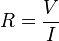$R = \frac{V}{I}$

where

R is the resistance of the object, measured in Ohms, equivalent to Js/C2
V is the potential difference across the object, measured in Volts
I is the current through the object, measured in Amperes

The ratio of voltage divided by electric current is also called the chordal resistance.

## Resistance of a conductor

### DC resistance

As long as the current density is totally uniform, the DC resistance R of a conductor of regular cross-section can be computed as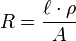$R = {\ell \cdot \rho \over A} \,$

where

is the length of the conductor, measured in meters
A is the cross-sectional area, measured in square meters
ρ (Greek: rho) is the electrical resistivity (also called specific electrical resistance) of the material, measured in Ohm • meter. Resistivity is a measure of the material's ability to oppose electric current.

For practical reasons, almost any connections to a real conductor will almost certainly mean the current density is not totally uniform. However, this formula still provides a good approximation for long thin conductors such as wires.

### AC resistance

If a wire conducts high-frequency alternating current then the effective cross sectional area of the wire is reduced because of the skin effect. This causes the wire resistance to increase at a rate of 10dB/decade for wire radius much greater than skin depth.

In a conductor close to others, the actual resistance is higher than that predicted by the skin effect because of the proximity effect.

## Causes of resistance

### In metals

A metal consists of a lattice of atoms, each with a shell of electrons. This can also be known as a positive ionic lattice. The outer electrons are free to dissociate from their parent atoms and travel through the lattice, creating a 'sea' of electrons, making the metal a conductor. When an electrical potential difference (a voltage) is applied across the metal, the electrons drift from one end of the conductor to the other under the influence of the electric field.

Near room temperatures, the thermal motion of ions is the primary source of scattering of electrons (due to destructive interference of free electron wave on non-correlating potentials of ions), and is thus the prime cause of metal resistance. Imperfections of lattice also contribute into resistance, although their contribution in pure metals is negligible.

The larger the cross-sectional area of the conductor, the more electrons are available to carry the current, so the lower the resistance. The longer the conductor, the more scattering events occur in each electron's path through the material, so the higher the resistance. Different materials also affect the resistance.

### In semiconductors and insulators

In metals, the Fermi level lies in the conduction band (see Band Theory, below) giving rise to free conduction electrons. However, in semiconductors the position of the Fermi level is within the band gap, approximately half-way between the conduction band minimum and valence band maximum for intrinsic (undoped) semiconductors. This means that at 0 Kelvin, there are no free conduction electrons and the resistance is infinite. However, the resistance will continue to decrease as the charge carrier density in the conduction band increases. In extrinsic (doped) semiconductors, dopant atoms increase the majority charge carrier concentration by donating electrons to the conduction band or accepting holes in the valence band. For both types of donor or acceptor atoms, increasing the dopant density leads to a reduction in the resistance. Highly doped semiconductors hence behave metallic. At very high temperatures, the contribution of thermally generated carriers will dominate over the contribution from dopant atoms and the resistance will decrease exponentially with temperature.

### In ionic liquids/electrolytes

In electrolytes, electrical conduction happens not by band electrons or holes, but by full atomic species (ions) traveling, each carrying an electrical charge. The resistivity of ionic liquids varies tremendously by the salt concentration—while distilled water is almost an insulator, salt water is a very efficient electrical conductor. In biological membranes, currents are carried by ionic salts. Small holes in the membranes, called ion channels, are selective to specific ions and determine the membrane resistance.

### Resistance of various materials

 Material Resistivity,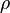$\rho$ ohm-meter Metals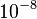$10^{-8}$ Semiconductors variable Electrolytes variable Insulators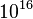$10^{16}$ Superconductors 0 (exactly)

### Band theory simplified

Quantum mechanics states that the energy of an electron in an atom cannot be any arbitrary value. Rather, there are fixed energy levels which the electrons can occupy, and values in between these levels are impossible. The energy levels are grouped into two bands: the valence band and the conduction band (the latter is generally above the former). Electrons in the conduction band may move freely throughout the substance in the presence of an electrical field.

In insulators and semiconductors, the atoms in the substance influence each other so that between the valence band and the conduction band there exists a forbidden band of energy levels, which the electrons cannot occupy. In order for a current to flow, a relatively large amount of energy must be furnished to an electron for it to leap across this forbidden gap and into the conduction band. Thus, even large voltages can yield relatively small currents.

## Differential resistance

When resistance may depend on voltage and current, differential resistance, incremental resistance or slope resistance is defined as the slope of the V-I graph at a particular point, thus:$R = \frac {\mathrm{d}V} {\mathrm{d}I} \,$

This quantity is sometimes called simply resistance, although the two definitions are equivalent only for an ohmic component such as an ideal resistor. For example, a diode is a circuit element for which the resistance depends on the applied voltage or current.

If the V-I graph is not monotonic (i.e. it has a peak or a trough), the differential resistance will be negative for some values of voltage and current. This property is often known as negative resistance, although it is more correctly called negative differential resistance, since the absolute resistance V/I is still positive. Example of such an element is a tunnel diode.

## Temperature-dependence

Near room temperature, the electric resistance of a typical metal varies linearly with the temperature. At lower temperatures (less than the Debye temperature), the resistance decreases as T5 due to the electrons scattering off of phonons. At even lower temperatures, the dominant scattering mechanism for electrons is other electrons, and the resistance decreases as T2. At some point, the impurities in the metal will dominate the behavior of the electrical resistance which causes it to saturate to a constant value. Matthiessen's Rule (first formulated by Augustus Matthiessen in the 1860s; the equation below gives its modern form) says that all these different types of behavior can be summed up to get the total resistance as a function of temperature,$R = R_\text{imp} + a T^2 + b T^5 + cT \,$

where Rimp is the temperature independent electrical resistivity due to impurities, and a, b, and c are coefficients which depend upon the metal's properties. This rule can be seen as the motivation to Heike Kamerlingh Onnes's experiments that lead in 1911 to the discovery of superconductivity. For details see History of superconductivity.

The electric resistance of a typical intrinsic (non doped) semiconductor decreases exponentially with the temperature:$R= R_0 e^{-aT}\,$

Extrinsic (doped) semiconductors have a far more complicated temperature profile. As temperature increases starting from absolute zero they first decrease steeply in resistance as the carriers leave the donors or acceptors. After most of the donors or acceptors have lost their carriers the resistance starts to increase again slightly due to the reducing mobility of carriers (much as in a metal). At higher temperatures it will behave like intrinsic semiconductors as the carriers from the donors/acceptors become insignificant compared to the thermally generated carriers.

The electric resistance of electrolytes and insulators is highly nonlinear, and case by case dependent, therefore no generalized equations are given.

## Measuring resistance

An instrument for measuring resistance is called a resistance meter or ohmmeter. Simple ohmmeters cannot measure low resistances accurately because the resistance of their measuring leads causes a voltage drop that interferes with the measurement, so more accurate devices use four-terminal sensing.